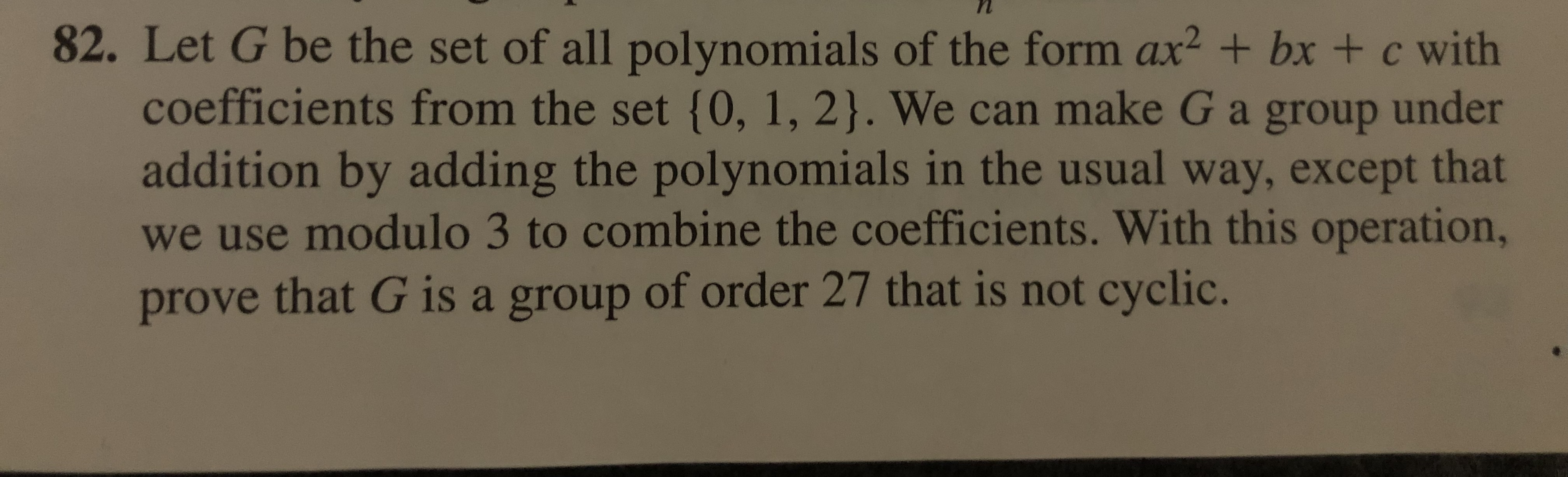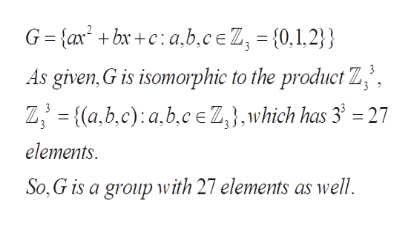# 82. Let G be the set of all polynomi als of the form ax2 + bx + c withcoefficients from the set {0, 1, 2}. We can make G a group underaddition by adding the polynomi als in the usual way, except thatwe use modulo 3 to combine the coefficients. With this operation,prove that G is a group of order 27 that is not cyclic.

Question
9 views

82help_outlineImage Transcriptionclose82. Let G be the set of all polynomi als of the form ax2 + bx + c with coefficients from the set {0, 1, 2}. We can make G a group under addition by adding the polynomi als in the usual way, except that we use modulo 3 to combine the coefficients. With this operation, prove that G is a group of order 27 that is not cyclic. fullscreen
check_circle

Step 1

To prove that (1) G is a group or order 27 and (2) G is not a cyclic group

Step 2

(1) By very definition, G is isomorphic to the Cartesian product gro...help_outlineImage TranscriptioncloseG {ax +bx+c: a,b,c e Z, = {0,1,2}} As given,G is isomorphic to the product Z', Z {(a,b,c) a,b,ceZ,},which has 3 =27 elements So, G is a group with 27 elements as well fullscreen

### Want to see the full answer?

See Solution

#### Want to see this answer and more?

Solutions are written by subject experts who are available 24/7. Questions are typically answered within 1 hour.*

See Solution
*Response times may vary by subject and question.
Tagged in

### Math